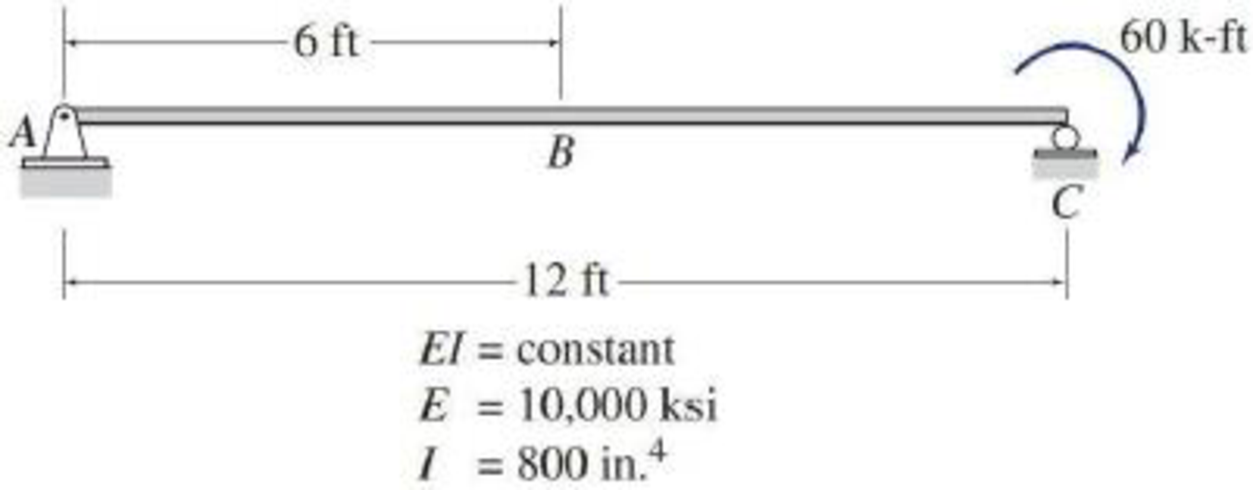# 6.7 and 6.8 Determine the slope and deflection at point B of the beam shown by the direct integration method. FIG. P6.8

#### Solutions

Chapter
Section
Chapter 6, Problem 8P
Textbook Problem
182 views

## 6.7 and 6.8 Determine the slope and deflection at point B of the beam shown by the direct integration method.FIG. P6.8To determine

Find the slope θB and deflection ΔB at point B of the given beam using the direct integration method.

### Explanation of Solution

Given information:

The Young’s modulus (E) is 10,000 ksi.

The moment of inertia (I) is 800in.4.

Calculation:

Consider downward as negative and upward as positive.

Show the free body diagram of the given beam as in Figure (1).

Refer Figure (1),

Determine the support reaction at C using the Equation of equilibrium.

MA=0RC×12+60=0RC=6012RC=5kips()

Determine the support reaction at A using the Equation of equilibrium.

V=0RA+RC=0RA=RCRA=5kips()

Equation for bending moment:

Take a section at a distance of x from support A.

Consider clockwise as negative and counterclockwise as positive.

Show the free body diagram of the given beam with the section as in Figure (2).

Refer Figure (2),

Writ the Equation for bending moment about the section x as follows:

Mx=RA×x=5x        (1)

Write the Equation for M/EI as follows:

d2ydx2=MEI        (2)

Substitute 5x for M in Equation (2).

d2ydx2=5xEI        (3)

Equation for slope and deflection:

The Equation for the slope of the elastic curve of the beam can be obtained by integrating the Equation for M/EI.

Integration Equation (3) and it can be written as follows:

θ=dydx=5EIx22+C1=5x22EI+C1        (4)

Integrate Equation (4), and it provides the Equation for deflection.

y=52EIx33+C1x+C2=5x36EI+C1x+C2        (5)

Express the boundary condition which is applied to evaluate the constants of integration as follows:

Atx=0,y=0

Atx=12ft,y=0

Apply the first boundary condition in Equation (5).

0=5(0)36EI+C1(0)+C2C2=0

Apply the second boundary condition in Equation (5).

0=5(12)36EI+C1(12)+0C1=8,640(12)6EIC1=120EI

Substitute 0 for C2 and 120EI for C1 in Equation (5).

y=5x36EI+120xEI+0=5x(x26EI+24EI)=5x6EI(x2+144)

Substitute 120EI for C1 in Equation (4)

### Still sussing out bartleby?

Check out a sample textbook solution.

See a sample solution

#### The Solution to Your Study Problems

Bartleby provides explanations to thousands of textbook problems written by our experts, many with advanced degrees!

Get Started

Find more solutions based on key concepts
Discuss in detail at least two methods or procedures that can be employed by airline companies to pick up your ...

Engineering Fundamentals: An Introduction to Engineering (MindTap Course List)

Write the script for a one-minute explanation of basic project management concepts.

Systems Analysis and Design (Shelly Cashman Series) (MindTap Course List)

Briefly describe a micro drill press and its purpose.

Precision Machining Technology (MindTap Course List)

Explain how a computer uses ports and connectors.

Enhanced Discovering Computers 2017 (Shelly Cashman Series) (MindTap Course List)

If your motherboard supports ECC DDR3 memory, can you substitute non-ECC DDR3 memory?

A+ Guide to Hardware (Standalone Book) (MindTap Course List)

What should be done if the torch flashes back?

Welding: Principles and Applications (MindTap Course List)